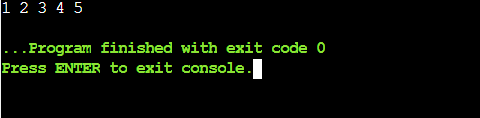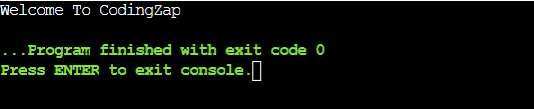# What Is Static Function In C++? (With Examples and Code)Are you curious to know about “Static Functions in C++“? Enter the world of C++ and meet the static functions. These incredible functions possess unique abilities that simplify your code and make it more efficient. In this article, we will discuss about the implementation process of static functions in C++.

But before we learn about the Static Function in C++ programming language, we have to learn static keywords & static variables in C++ programming language. So, we will gather all the necessary information related to the static function in the C++ programming language. So, let’s start our topic.

As usual, we will try to understand the necessity & the implementation process of the static function in C++ programming language using one daily life example. We will find out the necessity of using a static variable in C++ programming language first.

Then we will know about the static function in C++ programming language. Moreover, all the process is related to each other. If you are studying and stuck in your C++ Homework then you can use our C++ Homework Help Services.

## What Is Static In C++ Programming Language?

Static is a keyword in the C++ programming language that can be provided to a variable or a function to ‘fix’ its memory. This means that if something is defined as ‘static,’ its memory cannot be changed.As a keyword, the static can be placed before any objective in the C++ programming language. If the keyword is before the variable, it will be termed a Static Variable. If the keyword is placed before any function, then it will be considered a Static Function in C++ programming language. Let us try to understand both of them one by one.

• ### Static Variable:

When the static keyword is used before any variable, the variable becomes a static variable. Its functionality will be different from others. For a simple variable, it becomes a null variable after closing the function.

The variable will be removed from the memory space after the closing of any function to save the memory space. But in the case of static variables, the variable space still exists in the memory after the closing of the function.

It will help to implement some serious difficult issues. We will learn more about it when we implement one of these.

• ### Static Function:

Static function in C++ programming language is derived from the concept of the static variable. If the static keyword is used before the function name, it becomes a static function.

We used to write these things in Java programming language. But here, we need to intentionally declare a static function. If you’re learning Java, then you must be interested to know about the ways of converting an object to an integer.

The static function in C++ programming language can only able to access the static variables or other static functions declared under that said function. While accessing any non-static variable or function using any static function in C++, you will face some errors.

The static function in C++ programming language creates a copy of the member function to the other number of class objects.

• ### Static Variable Along Function:

Now, this can be considered the third category. Static functions in C++ programming language can also be defined into this category. Though, there is the least use of this category. But still, it is a valuable one.

It is a combination of both categories mentioned above. Here a static variable is developed. But it should work along with a function under a separate class. That static variable can be used outside of that class.

But while using that variable, the function declared under the class will also be used. So, one is dependent upon another. It will be easily understood when we provide one example.

Now, all categories where the static keyword is used are listed above. You might be getting confused about all the categories mentioned above. But there is no need to worry. We are here to clear all the doubts.

You will know more about them when we implement those using simple codes. We will implement the examples to clear the confusion related to it. Now, let us move forward to know static functions in the C++ programming language.

## What Is The Implementation Process Of Static Variable In C++?

The implementation process of a static variable in C++ programming language is moreover simple. Here, we need to first declare one function. It will be a simple function to work.

Under that function, we will declare one variable as static. We need to use a static keyword before the declaration of the variable. Then we have provided a value to it. Now, we are just increasing the variable with 1 & then we are printing it.

Now, in the main function, we need to declare one loop. That might be any loop as per your choice. Here, we have declared one while loop. The loop is calling the function for a certain amount of time. In normal cases, the variable declared under the function will print only the provided value. But in this case, as the variable is a static one, it will print the sequence of numbers. Here, the increment of the variable works as it is static.

General Syntax: Static data-type variable-name;

Code to implement static variable in C++:

```				```
#include <iostream>
#include <string>
using namespace std;

void codingzap()
{
static int zap = 0; // Static Variable Declaration

zap++; // Increment Of Variable

cout << zap << " ";
} // Printing Data Of The Static Variable

int main(){int i=0; while(i<5){ // While Loop Implementation

codingzap(); // Calling The Function

i++;}
return 0;}

```
```

Output:## How to Implement Static Data Members in C++?

A variable that is a part of a class is said to be a data member of a class. We can also provide the ‘static’ keyword to a data member or member variables of the class. Then, they become static data members. Let us understand this with an example.

To implement a static data member, we first need to create a class. Let’s create a class named CodingZap. Inside this class, let us declare a data member of data type int.

Now, we will make this data member static and also create a member function that will help us display the value of this data member. Also, using the scope resolution operator, we will initialize the value of the static data member outside the class.

Let us see the below code to understand how this works.

Code to implement static data members in C++:

```				```
#include <iostream>
using namespace std;

class codingZap {
public:
static int zap; // static data member

void display() {
cout << "Static Data Member Value: " << zap << endl;
}
};

// Initialization of the static data member outside the class definition
int codingZap::zap = 10;

int main() {
codingZap obj1, obj2;

// Accessing and displaying the static data member value using objects of the class
obj1.display();
obj2.display();
return 0;
}

```
```

Output:Here, we see that the static data member has the same value across all the objects of the class. If we modify the static data member using one object of the class, the change will also be reflected in other objects of the class whenever we access that particular static data member.

## How Implementation Of Static Function In Cpp Is Done?

Now, as we know the implementation process of a static variable in C++ programming language, it will be an easy task to implement the static function in C++ programming language.A static function in C++ belongs to a class. Like constructors, these are also evoked using the class name. A class in C++ consists of data members and member functions.

Both of these can be allocated the keyword ‘Static’ that helps to differentiate these class members from others. It also helps to define the scope of the class members.

Now, let us see how can we implement static member functions in C++.

For the implementation of the Static function in C++ programming language, we need to declare one class first. It is a simple class declaration.

Under that class, we need to declare one function. That function will be the static function in the C++ programming language. Before the function name, we need to prove the static keyword.

Under that function, we can write any statement. Like here, we are printing one message. Now, we need to conclude the class there. Then we need to proceed to the main function.

It is time to define the main function. In the main function, we will directly call the static function. Hence, it will execute all the statements mentioned there. We need to use the scope resolution operator (“::”) to call the function. The scope resolution operator should be used properly. The general syntax is provided for help. Using the class name & the function name, we can call the function.

General Syntax: class-name::function-name();

```				```
#include<iostream> using namespace std;

class zap{ // Class Declaration

public:

static void print(){ // Static Function

cout<<"Welcome To CodingZap";}};  // Statement Provided In Function

int main(){ zap::print(); // Calling Static Function

return 0;}

```
```

Let us try to find out the output of the above code. It will help to understand the static function in the C++ programming language.

Output:## What are the Properties of Static Member Functions in C++?

Now that we know how to implement a static member function in C++, let us move on to our next section and understand what are the properties of static member functions in C++.

Below are some of the key properties of static member functions-

• A static member function of a class belongs to a class itself and does not require an object of the class to invoke it.
• Static member functions can also be used to access other static members irrespective of their visibility, i.e. they can even access the private static members of a class.
• A scope resolution operator can be used to access a static member function.
• Static member functions can also be called even if no object of the class is created.
• Another key property of static member functions is that they lead to better memory efficiency as they do not require any object of the class.

## Conclusion:

As we saw, the static function in C++ programming language is an important topic.

We need to know the difference between the static variable & the static function in C++ programming language. Static is usually associated with classes in C++, hence, static variables can be turned into static data members (member variables) and a static function in C++ inside a class is said to be a static member function.

The second category is known as the implementation process of static functions in the C++ programming language. Other categories are used for background purposes.

It is advisable to clear the basic concept of the C++ programming language. Otherwise, there will be a knowledge gap. The implementation process of class & syntax writing of C++ programming language needs to be followed up.

It will help to know this topic in an easy & better process. It will help us in the future. Also, if you’re looking for C homework help, then you can contact us!

So, hope you have liked this piece of article. Share your thoughts in the comments section and let us know if we can improve more.